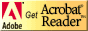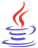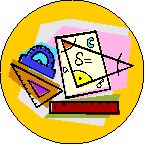# Standardized Test Preparation and Tips for Success

## Strand Resources: MeasurementCT4ME has identified resources related to each benchmark of the Ohio Mathematics Academic Content Standards that students should have mastered by the end of the grades 8-10 program.

Identify the benchmark (A-F) for review in Measurement.  Below the benchmark, you will find Web resources for reviewing the concept and practice problems.

Students should be able to review on their own using these resources.  If you are not an Ohio educator, these resources can still benefit your test prep efforts, as benchmarks in other states are similarly stated.

Measurement (pdf) This test prep booklet (about 200 KB) is designed to help you monitor your progress toward mastery of each benchmark in this strand.  You can print the entire booklet, or just those pages for benchmarks you need to work on.  Write notes in the booklet next to each resource you use in your review.If needed, download Adobe Acrobat Reader, free software for viewing and printing PDF files.You might need to download Java, a free plug-in to interact with virtual manipulatives on this page.Other strand resources:

Number, Number Sense, and Operations ]   [ Measurement ]   [ Geometry and Spatial Sense ]   [ Patterns, Functions, and Algebra ]   [ Data Analysis and Probability ]   [ Mathematical Processes ]### What is Measurement?### Benchmarks in Measurement

Benchmarks in this strand have been correlated to the Common Core State Standards (CCSS) for Mathematics for the Conceptual Category (CC), Domain (D), and Cluster (C).A.  Solve increasingly complex non-routine measurement problems and check for reasonableness of results.

CCSS: CC: Number and Quantity, D: Quantities, C: Reason quantitatively and use units to solve problems.

CCSS: CC: Geometry, D: Circles, C: Understand and apply theorems about circles.

CCSS: CC: grade 7, D: The Number System, C: Apply and extend previous understandings of operations with fractions to add, subtract, multiply, and divide rational numbers.

Approximations for Metric Conversion from National Institute of Standards and Technology, an agency of the US Dept. of Commerce:

ScienceSpot.net: Metric Mania includes lessons and worksheets with answers.Play the YouTube videos from the Ohio Resource Center Tutorials for High School Mathematics:

B.  Use formulas to find surface area and volume for specified three-dimensional objects accurate to a specified level of precision.

CCSS: CC: Geometry, D: Geometric Measurement and Dimension, C: Explain volume formulas and use them to solve problems.

CCSS: grade 8, D: Number System, C: Solve real-world and mathematical problems involving volume of cylinders, cones and spheres.

Math.Com, Prisms, Pyramids, Cylinders, Cones, Spheres: lesson and workout problems

Play the VideoMathTutor.com video (Free complete lesson):

• Algebra: Formulas From Geometry. Formulas and concepts covered include angles (complementary & supplementary), triangles (perimeter and area), isosceles triangle, equilateral triangle, right triangle, Pythagorean Theorem, similar triangles perimeter and area formulas for quadrilaterals (square, rectangle, parallelogram, trapezoid). Some diagonal formulas, too, where applicable are included. Circles (radius, diameter, circumference, area), cube (diagonal of face, diagonal of cube, surface area and volume), rectangular box [or rectangular parallelepiped] (diagonal of box, surface area and volume), prisms (volume), pyramids (volume), cone (slant height, lateral surface area, total surface area, volume), cylinder (lateral surface area, total surface area, volume), and sphere (surface area and volume) are then reviewed.

C.  Apply indirect measurement techniques, tools and formulas, as appropriate, to find perimeter, circumference and area of circles, triangles, quadrilaterals and composite shapes, and to find volume of prisms, cylinders, and pyramids.

CCSS: CC: Geometry, D: Geometric Measurement and Dimension, C: Explain volume formulas and use them to solve problems.

Math.Com: Polygon Basics, Triangles, Quadrilaterals, Area of Polygons and Circles lesson and workout problems

AlgebraLab.org:  Fill in the blank practice problems, including word problems.  Be sure to show the related AlgebraLab documents for lessons and additional practice problems on each topic.

D.  Use proportional reasoning and apply indirect measurement techniques, including right triangle trigonometry and properties of similar triangles, to solve problems involving measurements and rates.

CCSS: CC: Geometry, D: Similarity, Right Triangles, and Trigonometry, C: Define trigonometric ratios and solve problems involving right triangles.

CCSS: CC: Geometry, D: Circles, C: Understand and apply theorems about circles, and C: Find arc lengths and areas of sectors of circles.

E.  Estimate and compute various attributes, including length, angle measure, area, surface area and volume, to a specified level of precision.

CCSS: CC: Geometry, D: Geometric Measurement and Dimension, C: Explain volume formulas and use them to solve problems.

Mathguide.com:

• Area of Common Figures: rectangles, parallelograms, triangles, trapezoids, and circles.  Lesson and quizmasters.  Fill in and check answers.

• Volume and surface area for rectangular prisms, cylinders, square based pyramids, cones, spheres.  Note to Ohio learners: for spheres and cones, only find volume.  Quizmasters can be accessed from the main lesson page by scrolling down to Geometry: Three dimensional solids. Fill in and check answers.

Math Open Reference: Use virtual manipulatives to explore:

GeoGebra: Polygons and Angles: A series of interactive applets created with GeoGebra for exploring interior and exterior angles of polygons.

F.  Write and solve real-world, multi-step problems involving money, elapsed time and temperature, and verify reasonableness of solutions.

CCSS: CC: grade 7, D: Ratio and Proportionality Relationships, C: Analyze proportional relationships and use them to solve real-world and mathematical problems.

CCSS: CC: grade 7, D: The Number System, C: Apply and extend previous understandings of operations with fractions to add, subtract, multiply, and divide rational numbers.Are you ready for the test?

#### References

*Ohio Mathematics Academic Content Standards (2001). http://www.ode.state.oh.us/GD/Templates/Pages/ODE/ODEPrimary.aspx?page=2&TopicID=1696&TopicRelationID=1704 [URL is no longer active.]

Ohio Mathematics Academic Content Standards Crosswalk, K-8 and High School (2010).  https://web.archive.org/web/20180206120934/http://education.ohio.gov/getattachment/Topics/Ohio-s-New-Learning-Standards/Mathematics/Transition-Tools-Ohio-s-New-Learning-Standards-K-1/Alignment-Toolkit-Phase-1-Gap-Analysis/HS-crosswalk-mathematics-4-17-12.pdf.aspx [Revised 4/17/2012].

*Note 2019: The current academic content standards for mathematics are now available at http://education.ohio.gov/Topics/Learning-in-Ohio/Mathematics/Ohio-s-Learning-Standards-in-MathematicsSee other strand resources:

Number, Number Sense, and Operations ]   [ Measurement ]   [ Geometry and Spatial Sense ]   [ Patterns, Functions, and Algebra ]   [ Data Analysis and Probability ]   [ Mathematical Processes ]See related pages: [ Standardized Test Preparation and Tips for Success (first  page)]  [ OGT Introduction ]